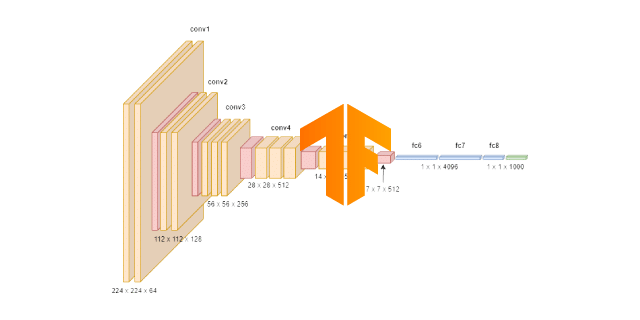#### Examples

Full code examples that you can use as a starting point for your projects.Spam detection

Build deep learning model using feed forward neural network to classify emails into two categories – spam or not-spam.

binary classification feed forward neural networkCredit card fraud detection

Build deep learning model using feed forward neural network to classify credit card transactions into two categories – fraud or not-fraud

binary classification feed forward neural networkSonar signal classification

Build deep learning model using feed forward neural network to classify sonar signals to detect mines or rocks.

binary classification feed forward neural networkMulti class classification

Build machine learning model to classify Iris Flowers using Feed Forward neural network and data from CSV file.

classification feed forward neural networkSimple linear regression

Build machine learning model to approximate noisy data that have uniform ascending or descending (linear trend).

regressionfeed forward neural networkInsurance payment prediction

Build deep learning model using feed forward neural network to predict total payments for auto insurance given the number of claims.

regression feed forward neural networkCPU performance prediction

Build ML model to predict CPU performance using data from CSV file.

regressionfeed forward neural networkHouse price prediction

Build machine learning model to predict house price based on data from CSV file.

regressionfeed forward neural networkHot Dog or Not Hot Dog

Build deep learning model using convolutional neural network to classify images into ‘hot dog’ and ‘not hot dog’ categories.

convolutional neural networkimage recognitionDuke Logo Recognition

Build deep learning model using convolutional neural network to recognize Java Duke logo images.

convolutional neural networkimage recognitionParking Lot Occupancy

Build deep learning model to create visual parking lot occupancy detection using convolutional network in Java.

convolutional neural networkimage recognitionLego Figures Recognition

Build deep learning model using convolutional neural network to recognize Lego figures.

convolutional neural networkimage recognitionHandwritten digit recognition

Build deep learning model in Java to recognize handwritten digits using convolutional neural network

convolutional neural networkimage recognitionVggNet16 Tensorflow Import

Import large convolutional neural network VGGNet16 trained in Tensorflow into Deep Netts and use it to recognize 1000 different objects

convolutional neural networkimage recognition